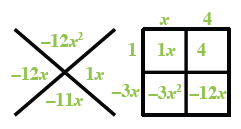### Home > CCA2 > Chapter 3 > Lesson 3.1.2 > Problem3-27

3-27.

For the function $h(x) = −3x^2 − 11x + 4$, find the value of $h(x)$ for each value of $x$ given below.

1. $h(0)$

Substitute $0$ for $x$ and simplify.

$h(0)=−3(0)2−11(0)+4$

$h(0)=4$

1. $h(2)$

See part (a).

$h(2)=−30$

1. $h(−1)$

Be careful of the signs when multiplying negative numbers.

$h(−1)=12$

1. $h\left(\frac{1}{2}\right)$

Remember how to multiply fractions.

1. For what value(s) of $x$ does $h(x)=0$

$0=−3x^2−11x+4$